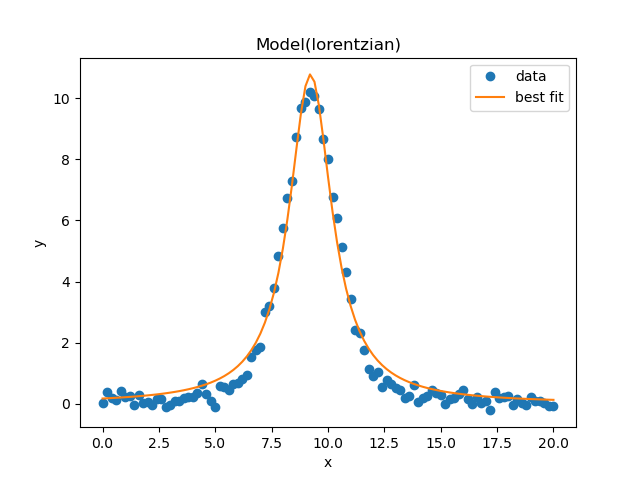# Fit with Data in a pandas DataFrame¶

Simple example demonstrating how to read in the data using pandas and supply the elements of the DataFrame from lmfit.

import matplotlib.pyplot as plt
import pandas as pd

from lmfit.models import LorentzianModel


read the data into a pandas DataFrame, and use the ‘x’ and ‘y’ columns:

dframe = pd.read_csv('peak.csv')

model = LorentzianModel()
params = model.guess(dframe['y'], x=dframe['x'])

result = model.fit(dframe['y'], params, x=dframe['x'])


and gives the plot and fitting results below:

result.plot_fit()
plt.show()

print(result.fit_report())Out:

[[Model]]
Model(lorentzian)
[[Fit Statistics]]
# fitting method   = leastsq
# function evals   = 21
# data points      = 101
# variables        = 3
chi-square         = 13.0737250
reduced chi-square = 0.13340536
Akaike info crit   = -200.496119
Bayesian info crit = -192.650757
[[Variables]]
amplitude:  39.1530829 +/- 0.62389698 (1.59%) (init = 50.7825)
center:     9.22379520 +/- 0.01835869 (0.20%) (init = 9.3)
sigma:      1.15503893 +/- 0.02603688 (2.25%) (init = 1.3)
fwhm:       2.31007785 +/- 0.05207377 (2.25%) == '2.0000000*sigma'
height:     10.7899514 +/- 0.17160472 (1.59%) == '0.3183099*amplitude/max(1e-15, sigma)'
[[Correlations]] (unreported correlations are < 0.100)
C(amplitude, sigma) =  0.709


Total running time of the script: ( 0 minutes 0.385 seconds)

Gallery generated by Sphinx-Gallery